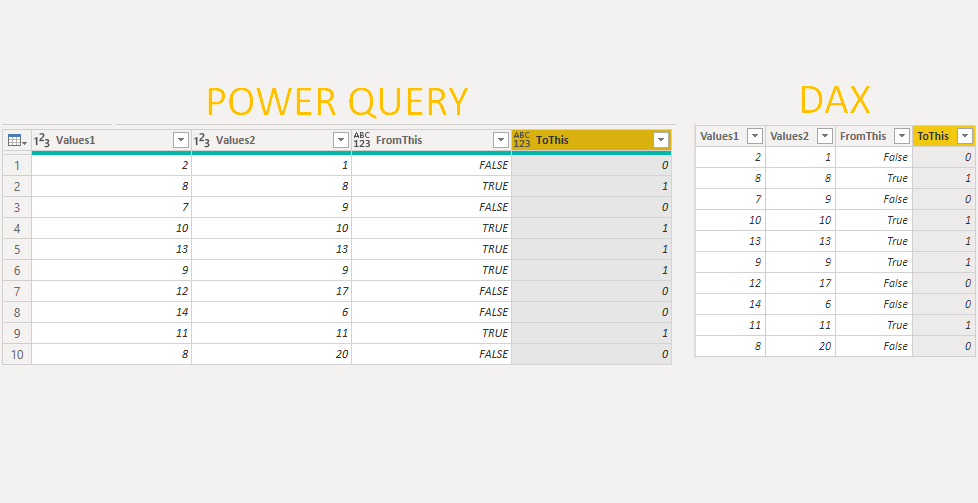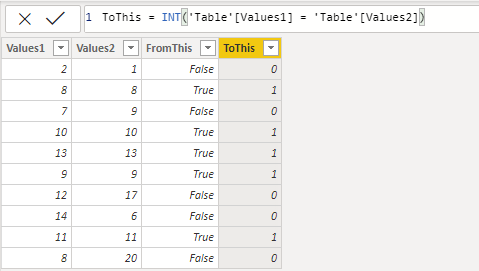# Convert TRUE and FALSE into 1 and 0 in Power BIHere is a simple way how to convert TRUE and FALSE into 1 and 0 in Power BI. Precisely speaking – Power Query and DAX.

## Power Query

To convert TRUE and FALSE into 1 and 0 use Number.From.

`=Number.From([Values1]=[Values2])`Here are other Power Query posts that might be interesting for you.

## DAX

To convert TRUE and FALSE into 1 and 0, use INT function or multiplay with 1.

`ToThis = INT('Table'[Values1] = 'Table'[Values2])`Here are other DAX posts that might be interesting for you.